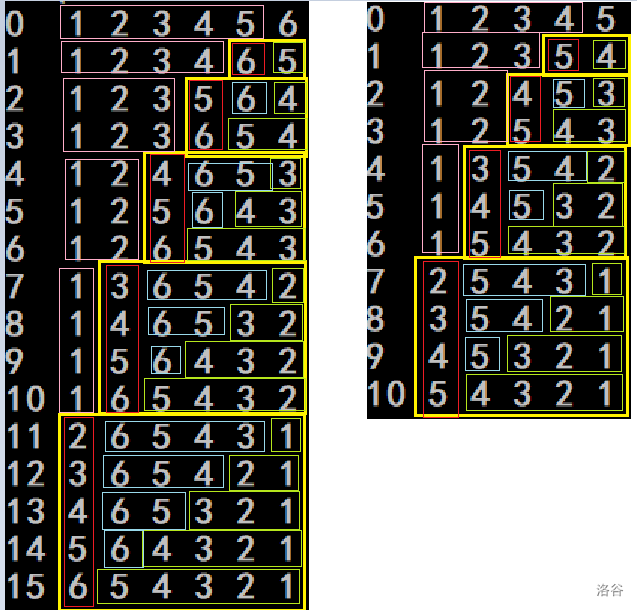2020-02-03 17:18:51

# 咱这规律找滴彳亍不彳亍#include<iostream>
#include<cstdio>
using namespace std;
int n,m;
int main(){
cin>>n>>m;
int b=0;
for(b=0;b<n && m>b;++b)  m-=b;
for(int i=1;i<=n-b-1;++i)  printf("%d ",i);
printf("%d ",n-(b-m));
for(int i=1,j=n;i<=b-m;++i,--j)  printf("%d ",j);
for(int i=1,j=n-(b-m)-1;i<=m;++i,--j)  printf("%d ",j);
cout<<endl;
return 0;
}

• star
首页